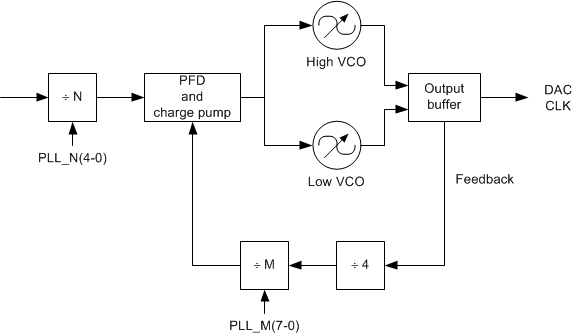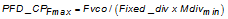SLASEA6D February   2017  – June 2020

PRODUCTION DATA.

1. Features
2. Applications
3. Description
4. Revision History
5. Device Comparison Table
6. Pin Configuration and Functions
7. Specifications
8. Detailed Description
1. 8.1 Overview
2. 8.2 Functional Block Diagrams
3. 8.3 Feature Description
4. 8.4 Device Functional Modes
5. 8.5 Register Maps
9. Application and Implementation
1. 9.1 Application Information
2. 9.2 Typical Application
10. 10Power Supply Recommendations
11. 11Layout
12. 12Device and Documentation Support
13. 13Mechanical, Packaging, and Orderable Information

• AAV|144

#### 8.4.3 Internal PLL/VCO

The DAC38RF82 (or DAC38RF89) has an internal clock generation circuit consisting of a PLL and two selectable VCOs, as shown in Figure 49.Figure 49. Internal PLL/VCO Block Diagram

DAC38RF82 (or DAC38RF89) each have a low VCO (VCO0) and high VCO (VCO1), but they are tuned to different center frequencies in each device. The VCO frequency ranges for each device are summarized in Electrical Characteristics - Digital Specifications. The VCO is selected through field PLL_VCOSEL in register PLL_CONFIG2 (8.5.81), with ‘0’ selecting the low VCO and a ‘1’ the high VCO. The 7 bit VCO tuning code in field PLL_VCO in register PLL_CONFIG2 (8.5.81) is used to tune the VCO frequency from the lowest frequency in the range to the highest frequency for the particular VCO used. For the low VCO the center VCO frequency is achieved with PLL_VCO = 63decimal and for the high VCO the center frequency is achieved with PLL_VCO = 63decimal.

The supply current, and therefore; the analog signal amplitude in the VCO is controlled using the field PLL_VCO_RDAC in register PLL_CONFIG1 (8.5.80). This control signal should be set 15decimal initially for 18 mA supply current in the VCO and ~1.4 VPP single ended oscillation amplitude.

The PLL has no prescaler, so the DAC sample rate is the VCO frequency. In the PLL feedback path a fixed ÷ 4 frequency divider block receives the VCO output clock and divides its frequency by 4. The maximum operating frequency of the phase-frequency detector (PFD) and charge pump (CP) requires this. The M (feedback) clock divider takes the output clock signal from the fixed ÷4 block and divides it by a programmable ratio set by the 8-bit field in field PLL_M_M1 in register PLL_CONFIG1 (8.5.80). The programmable division ratio range is ÷1 to ÷256, and is the value of the 8 bit unsigned binary code + 1. Although it is possible to program the M divider to ÷1, ÷2 and ÷3, these values should not be used. As stated previously the PFD and CP have a finite maximum operating frequency, which is the VCO frequency divided by the fixed divider ratio multiplied by the minimum allowable M divider ratio.

Equation 18.The N (reference) divider determines the ratio between the input reference clock frequency and the PFD operating frequency, and is set by the 5-bit field PLL_N_M1 in register CLK_PLL_CFG (8.5.79). The division ratio range is ÷1 to ÷32, and is the value of the 5-bit unsigned binary code + 1.

The charge pump output current amplitude is set using the 4-bit field PLL_CP_ADJ in in register PLL_CONFIG2 (8.5.81). The current amplitude is simply the digital code multiplied by the unit current amplitude of 100 µA. In a nominal condition, with the DAC38RF82 VCO0 running at 5.898 GHz, and with the M divider set to ÷4, the PFD will run at 368.625 MHz, and the change pump current should set to 6decimal, which gives 600 µA charge pump output current for a good phase margin of 69 degrees. If a higher M ratio (for lower PFD frequencies) are required the charge pump output current must be increased to maintain good loop stability and prevent excessive peaking in the phase noise response. The charge pump output current setting PLL_CP_ADJ should be adjusted in relation to the feedback (M) divider ratio PLL_M_M1 according to the following table to maintain a constant phase margin of 69 degrees.